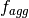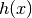# Overview on extracted features¶

tsfresh calculates a comprehensive number of features. All feature calculators are contained in the

 `tsfresh.feature_extraction.feature_calculators` This module contains the feature calculators that take time series as input and calculate the values of the feature.

submodule.

The following, exhaustive list contains all features that are calculated in the current version of tsfresh:

 `abs_energy`(x) Returns the absolute energy of the time series which is the sum over the squared values `absolute_sum_of_changes`(x) Returns the sum over the absolute value of consecutive changes in the series x `agg_autocorrelation`(x, param) Calculates the value of an aggregation function(e.g. `agg_linear_trend`(x, param) Calculates a linear least-squares regression for values of the time series that were aggregated over chunks versus the sequence from 0 up to the number of chunks minus one. `approximate_entropy`(x, m, r) Implements a vectorized Approximate entropy algorithm. `ar_coefficient`(x, param) This feature calculator fits the unconditional maximum likelihood of an autoregressive AR(k) process. `augmented_dickey_fuller`(x, param) The Augmented Dickey-Fuller test is a hypothesis test which checks whether a unit root is present in a time series sample. `autocorrelation`(x, lag) Calculates the autocorrelation of the specified lag, according to the formula  `benford_correlation`(x) Useful for anomaly detection applications . `binned_entropy`(x, max_bins) First bins the values of x into max_bins equidistant bins. `c3`(x, lag) This function calculates the value of `change_quantiles`(x, ql, qh, isabs, f_agg) First fixes a corridor given by the quantiles ql and qh of the distribution of x. `cid_ce`(x, normalize) This function calculator is an estimate for a time series complexity  (A more complex time series has more peaks, valleys etc.). `count_above`(x, t) Returns the percentage of values in x that are higher than t `count_above_mean`(x) Returns the number of values in x that are higher than the mean of x `count_below`(x, t) Returns the percentage of values in x that are lower than t `count_below_mean`(x) Returns the number of values in x that are lower than the mean of x `cwt_coefficients`(x, param) Calculates a Continuous wavelet transform for the Ricker wavelet, also known as the “Mexican hat wavelet” which is `energy_ratio_by_chunks`(x, param) Calculates the sum of squares of chunk i out of N chunks expressed as a ratio with the sum of squares over the whole series. `fft_aggregated`(x, param) Returns the spectral centroid (mean), variance, skew, and kurtosis of the absolute fourier transform spectrum. `fft_coefficient`(x, param) Calculates the fourier coefficients of the one-dimensional discrete Fourier Transform for real input by fast `first_location_of_maximum`(x) Returns the first location of the maximum value of x. `first_location_of_minimum`(x) Returns the first location of the minimal value of x. `fourier_entropy`(x, bins) Calculate the binned entropy of the power spectral density of the time series (using the welch method). `friedrich_coefficients`(x, param) Coefficients of polynomial, which has been fitted to `has_duplicate`(x) Checks if any value in x occurs more than once `has_duplicate_max`(x) Checks if the maximum value of x is observed more than once `has_duplicate_min`(x) Checks if the minimal value of x is observed more than once `index_mass_quantile`(x, param) Those apply features calculate the relative index i where q% of the mass of the time series x lie left of i. `kurtosis`(x) Returns the kurtosis of x (calculated with the adjusted Fisher-Pearson standardized moment coefficient G2). `large_standard_deviation`(x, r) Boolean variable denoting if the standard dev of x is higher than ‘r’ times the range = difference between max and min of x. `last_location_of_maximum`(x) Returns the relative last location of the maximum value of x. `last_location_of_minimum`(x) Returns the last location of the minimal value of x. `lempel_ziv_complexity`(x, bins) Calculate a complexity estimate based on the Lempel-Ziv compression algorithm. `length`(x) Returns the length of x `linear_trend`(x, param) Calculate a linear least-squares regression for the values of the time series versus the sequence from 0 to length of the time series minus one. `linear_trend_timewise`(x, param) Calculate a linear least-squares regression for the values of the time series versus the sequence from 0 to length of the time series minus one. `longest_strike_above_mean`(x) Returns the length of the longest consecutive subsequence in x that is bigger than the mean of x `longest_strike_below_mean`(x) Returns the length of the longest consecutive subsequence in x that is smaller than the mean of x `max_langevin_fixed_point`(x, r, m) Largest fixed point of dynamics :math:argmax_x {h(x)=0}` estimated from polynomial, `maximum`(x) Calculates the highest value of the time series x. `mean`(x) Returns the mean of x `mean_abs_change`(x) Returns the mean over the absolute differences between subsequent time series values which is `mean_change`(x) Returns the mean over the differences between subsequent time series values which is `mean_second_derivative_central`(x) Returns the mean value of a central approximation of the second derivative `median`(x) Returns the median of x `minimum`(x) Calculates the lowest value of the time series x. `number_crossing_m`(x, m) Calculates the number of crossings of x on m. `number_cwt_peaks`(x, n) This feature calculator searches for different peaks in x. `number_peaks`(x, n) Calculates the number of peaks of at least support n in the time series x. `partial_autocorrelation`(x, param) Calculates the value of the partial autocorrelation function at the given lag. `percentage_of_reoccurring_datapoints_to_all_datapoints`(x) Returns the percentage of non-unique data points. `percentage_of_reoccurring_values_to_all_values`(x) Returns the percentage of values that are present in the time series more than once. `permutation_entropy`(x, tau, dimension) Calculate the permutation entropy. `quantile`(x, q) Calculates the q quantile of x. `range_count`(x, min, max) Count observed values within the interval [min, max). `ratio_beyond_r_sigma`(x, r) Ratio of values that are more than r*std(x) (so r sigma) away from the mean of x. `ratio_value_number_to_time_series_length`(x) Returns a factor which is 1 if all values in the time series occur only once, and below one if this is not the case. `sample_entropy`(x) Calculate and return sample entropy of x. `set_property`(key, value) This method returns a decorator that sets the property key of the function to value `skewness`(x) Returns the sample skewness of x (calculated with the adjusted Fisher-Pearson standardized moment coefficient G1). `spkt_welch_density`(x, param) This feature calculator estimates the cross power spectral density of the time series x at different frequencies. `standard_deviation`(x) Returns the standard deviation of x `sum_of_reoccurring_data_points`(x) Returns the sum of all data points, that are present in the time series more than once. `sum_of_reoccurring_values`(x) Returns the sum of all values, that are present in the time series more than once. `sum_values`(x) Calculates the sum over the time series values `symmetry_looking`(x, param) Boolean variable denoting if the distribution of x looks symmetric. `time_reversal_asymmetry_statistic`(x, lag) This function calculates the value of `value_count`(x, value) Count occurrences of value in time series x. `variance`(x) Returns the variance of x `variance_larger_than_standard_deviation`(x) Boolean variable denoting if the variance of x is greater than its standard deviation. `variation_coefficient`(x) Returns the variation coefficient (standard error / mean, give relative value of variation around mean) of x.